Water Content - Moisture Content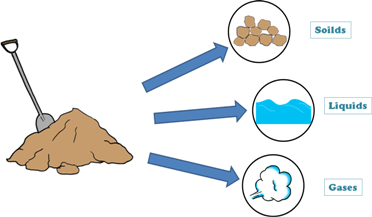A soil mass in general is composed of three kinds of matter,

1. Solids

2. Liquid

3. Gaseous

Solids are of different sizes and shapes and dur to which they enclose empty spaces in them. These empty spaces are called pores or voids.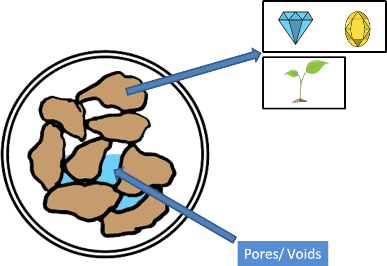These voids in the soil mass are either filled by liquid, which is generally water, or by gases, which is usually air, or they are filled by both, partly by water and partly by air. If voids are not occupied by the water then they are occupied by the air.

The proportions of solids, water and air in a soil mass influence its physical properties. And before founding any structure on the soil we should study the soil in detail including its physical properties.

For determining physical properties of soil we need to learn few simple terms which are frequently used in soil engineering.Let's begin with water content which is also called moisture content. Moisture is water.

It is denoted by small letter w.

Water content of any soil sample is defined as the ratio of weight of water to the weight of solids present in that sample. We also multiply it by hundred because it is usually expressed as percentage.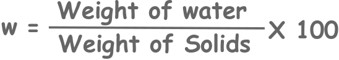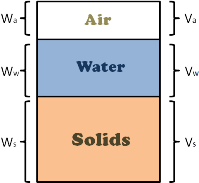let’s consider the phase diagram of a soil sample. Here weight of water is taken as Ww and weight of solids is Ws.

So formula becomes: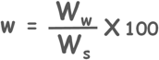So how do we determine the water content in a soil sample?

Well there are numerous methods to determine the water content of a soil.

Lets discuss a small soil mechanics problem and calculate water content of the soil sample.

Question: We are given a 1 cubic meter of wet soil which weights 20 KN.

Its dry weight is 18 KN.

We need to determine the water content of the given soil sample.

Solution: So here we are given with weight of wet soil 20 KN.

We know that weight of wet soil is equal to weight of solids plus weight of water.

And it is also given that weight of dry soil is 18 KN.

And we know that weight of solids is equal to weight of dry soil because weight of water is equal to zero and voids are only filled with the air and we have learned that we assume weight of air as zero.

So we can know the weight of water in the wet soil as weight of water is equal to weight of wet soil minus weight of dry soil

so weight of water comes as 2 KN

and by the formula we can calculate the water content of the soil sampleNow as we know that for dry soil, water content is 0%, which is the minimum water content possible in any soil.

But it has no upper limit. We can have as much water into soil as we want. But water content like 700% or 800% won’t be a soil sample, perhaps. It will be a water sample with soil as impurity.

Tags : water content, moisture content

Published on :2019-08-04